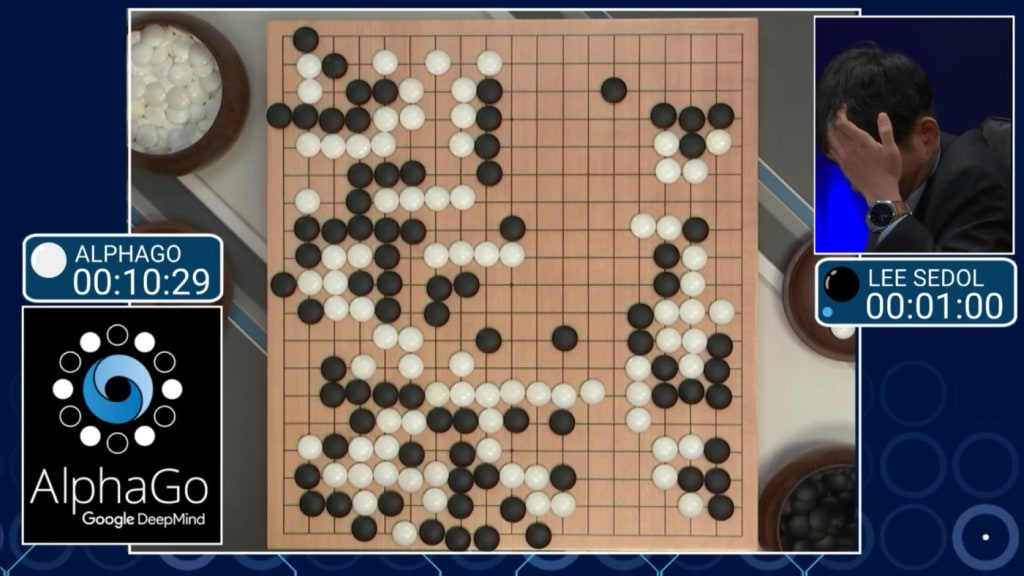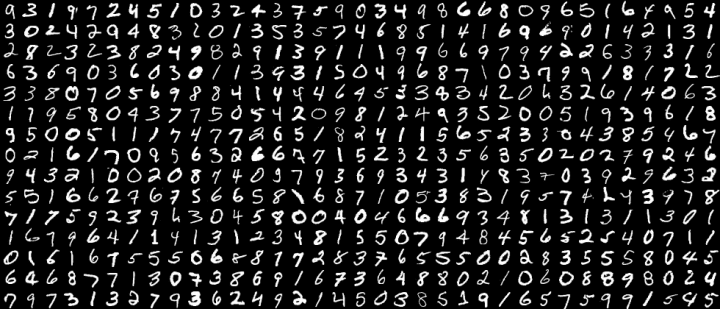# Classify Machine Learning algorithms

by Edward Nguyen 3 months ago in history

### There are two common ways of grouping Machine learning algorithms. One is based on the learning style, the other is based on the function (of each algorithm).1. Grouping based on learning style

According to the learning style, Machine Learning algorithms are usually divided into 4 groups: Supervise learning, Unsupervised learning, Semi-supervised learning, and Reinforcement learning. There are several subgroups that do not have Semi-supervised learning or Reinforcement learning.

Supervised Learning (Supervised Learning)

Supervised learning is an algorithm that predicts the outcome of a new input based on known pairs (input, outcome). This data pair is also known as (data, label), ie (data, label). Supervised learning is the most common group in Machine Learning algorithms.

Mathematically, supervised learning is when we have a set of input variables X={x1,x2,…,xN} and a corresponding set of labels Y={y1,y2,…,yN}, with

xi, yi are vectors. Pairs of known data are called training data sets. From this training data set, we need to create a mapping function for each element from the set X to a corresponding (approximate) element of the set Y

yi≈f(xi), ∀i=1,2,…,N

The goal is to approximate the function f so well that when there is a new data x, we can compute its corresponding label y = f (x).

Example 1: in handwriting recognition, we have pictures of thousands of examples of each digit written by different people. We put these images into an algorithm and show it which digit each image represents. After the algorithm creates a model, that is, a function whose input is a photograph and the output is a digit, when it receives a new image the model has never seen, it will predict the picture. What digit does that image contain?This example is quite similar to the way people learned in childhood. We give the alphabet to a child and show them this is the letter A, this is the letter B. After a few times of teaching, they can recognize what is A and which is B in a book they have never seen.

Example 2: The algorithm to detect faces in an image has been developed for a long time. At first, Facebook used this algorithm to identify the faces in a photo and ask users to tag friends - ie assign a label to each face. The greater the number of data pairs (faces, names), the greater the accuracy at subsequent auto-tagging.

Example 3: The algorithm to detect faces in an image is also a Supervised learning algorithm with training data of thousands of pairs (photos, faces) and (photos, not faces) ) is included. Note that this data only distinguishes human and non-human faces without distinguishing the faces of different people.

The supervised learning algorithm is further broken down into two main categories:

Classification

A problem is called classification if the labels of input data are divided into a finite group number. For example, Gmail determines whether an email is a spam or not; Credit firms determine whether a customer is able to pay off debt. The above three examples are divided into this category.

Regression

If the label is not divided into groups but rather a specific real value. For example, How much for a house with x (m ^ 2), y bedroom(s) and a distance of z km from the city center?

Microsoft recently had a face and age prediction app. The gender prediction part can be considered as the Classification algorithm, the age prediction part can be considered the Regression algorithm. Note that the age prediction can also be considered Classification if we consider age as a positive integer not greater than 150, we will have 150 different classes.

Unsupervised Learning (Unattended Learning)

In this algorithm, we do not know the outcome or label but only the input data. The unsupervised learning algorithm will rely on the structure of the data to perform a certain task, such as clustering or dimension reduction to facilitate storage and calculation.

Mathematically, Unsupervised learning is when we only have input to X without knowing the corresponding Y label.

Such algorithms are called Unsupervised learning because unlike Supervised learning, we don't know the correct answer for each input. Like when we study, no teacher will tell us whether it is an A or a B. An unattended cluster is named in this sense.

Unsupervised learning problems are further broken down into two categories:

Clustering

A problem that divides all X data into small groups based on the relationship between the data in each group. For example, grouping customers based on buying behavior. This is like giving a child lots of pieces of different shapes and colors, such as triangles, squares, and circles with blue and red, then asking them to divide them into groups... Although it is not possible for a child to tell which piece corresponds to which image or color, it is more likely that they can still classify the pieces by color or shape.

Association

The problem when we want to discover a rule based on a lot of given data. For example, male customers who buy clothes tend to buy more watches or belts; Spider-Man moviegoers tend to watch more Bat Man movies, which in turn creates a Recommendation System, which drives shopping demand.

Semi-Supervised Learning (Semi-Supervised Learning)

The problem when we have a large amount of X data but only part of them is labeled is called Semi-Supervised Learning. The problems in this group lie between the two groups listed above.

A typical example of this group is that only a portion of the photo or text is labeled (for example, photographs of people, animals or scientific or political texts) and most other photos/texts. Unlabelled labels are collected from the internet. In fact, many Machine Learning problems fall into this category because collecting branded data is time-consuming and expensive. Many types of data even require specialist labeling. In contrast, unlabeled data can be collected at low cost from the internet.

Reinforcement Learning

Reinforcement learning is the math that helps a system to automatically identify behavior based on circumstances to maximize the benefits (maximizing the performance). Currently, Reinforcement learning is mainly applied to Game Theory, the algorithms need to determine the next move to achieve the highest score.

2. Classify based on function

There is a second way of grouping based on the function of algorithms. In this section, I would only list the algorithms. Specific information will be presented in other posts on this publication(I will update asap). During the writing process, I might add or remove some algorithms.

Regression Algorithms

Linear Regression

Logistic Regression

Stepwise Regression

Classification Algorithms

Linear Classifier

Support Vector Machine (SVM)

Kernel SVM

Sparse Representation-based classification (SRC)

Instance-based Algorithms

k-Nearest Neighbor (kNN)

Learning Vector Quantization (LVQ)

Regularization Algorithms

Ridge Regression

Least Absolute Shrinkage and Selection Operator (LASSO)

Least-Angle Regression (LARS)

Bayesian Algorithms

Naive Bayes

Gaussian Naive Bayes

Clustering Algorithms

k-Means clustering

k-Medians

Expectation-Maximization (EM)

Artificial Neural Network Algorithms

Perceptron

Softmax Regression

Multi-layer Perceptron

Back-Propagation

Dimensionality Reduction Algorithms

Principal Component Analysis (PCA)

Linear Discriminant Analysis (LDA)

Ensemble Algorithms

Boosting

Random Forest

And there are many other algorithms.

3. References

A Tour of Machine Learning Algorithms

historyEdward Nguyen